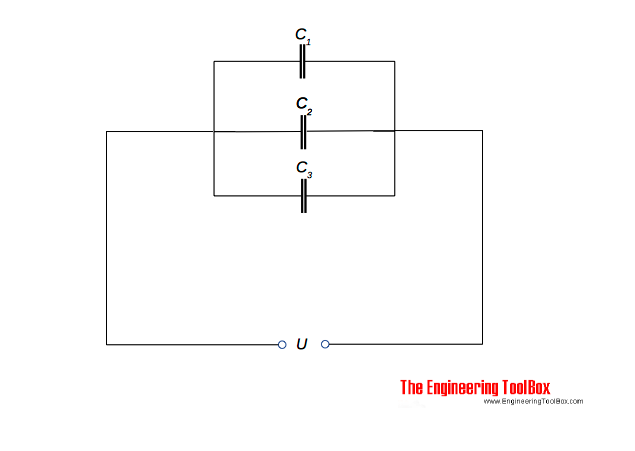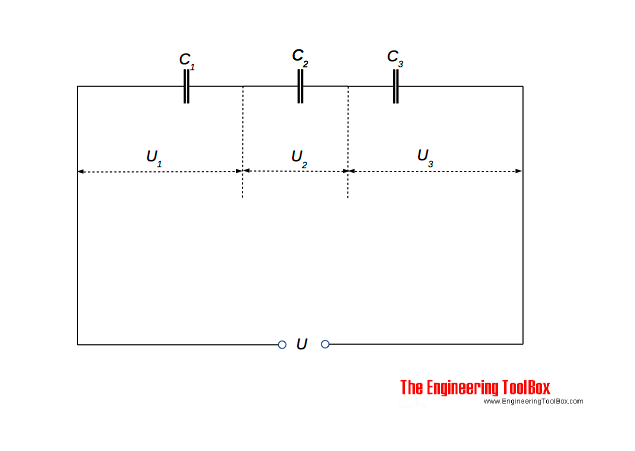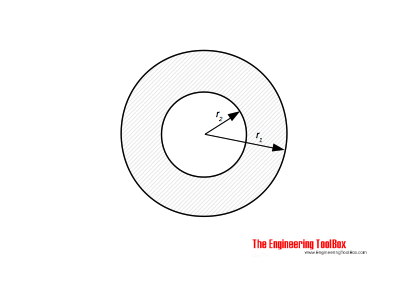Engineering ToolBox - Resources, Tools and Basic Information for Engineering and Design of Technical Applications!

# Capacitors - Parallel and Serial Connections

## Parallel and serial connected capacitor circuits.

### Capacitors in Parallel

Capacitors can be connected in parallel:The equivalent capacitance for parallel-connected capacitors can be calculated as

C = C1 + C2 + . . + Cn                     (1)

where

C = equivalent capacitance for the parallel connected circuit  (Farad, F, μF)

C1..n = capacitance capacitors (Farad, F, μF)

It is common to use µF as the unit for capacitance.

### Capacitors in Series

Capacitors can be connected in series:The equivalent capacitance for series-connected capacitors can be calculated as

1 / C = 1 / C1 + 1 / C2 + . . + 1 / Cn                  (2)

For the special case with two capacitors in series - the capacitance can be expressed as

1 / C = (C1 + C2) / (C1 C2)                     (2b)

- or transformed to

C = C1 C2 / (C1 + C2)                     (2c)

### Example - Capacitors Connected in Parallel and in Series

The equivalent capacitance of two capacitors with capacitance 10 μF and 20 μF  can be calculated as

in parallel

C = (10 μF) + (20 μF)

= 30 (μF)

in series

1 / C = 1 / (10 μF) + 1 / (20 μF)

= 0.15 (1/μF)

or

C = 1 / 0.15 (1/μF)

= 6.7 (μF)

### Capacitors in Series

Three capacitors C1 = 3 μF , C2 = 6 μF and C3 = 12 μF are connected in series as indicated in the figure above. The voltage supply to the circuit is 230 V.

The equivalent circuit capacitance can be calculated with (2)

1 / C = 1 / (3 μF) + 1 / (6 μF) + 1 / (12 μF)

= (4 + 2 + 1) / 12

= 0.58 1/μF

- or transformed

C = 12 / (4 + 2 + 1)

= 1.7 μF

The total charge in the circuit can be calculated with

Q = U C

where

Q = charge (coulomb, C)

U = electric potential (V)

- or with values

Q = (230 V) (1.7 10-6 F)

= 3.91 10-4 C

= 391 μC

Since the capacitors are connected in series - the charge is 391 μC on each of them.

The voltage across capacitor 1 can be calculated

U1 = Q / C1

= (391 μC) / (3 μF)

= 130 V

The voltage across capacitor 2 can be calculated

U2 = Q / C2

= (391 μC) / (6 μF)

= 65 V

The voltage across capacitor 3 can be calculated

U3 = Q / C3

= (391 μC) / (12 μF)

= 33 V

### Capacitance of Two Coaxial CylindersThe capacitance of two coaxial cylinders as indicated in the figure can be calculated as

C = 2 π εo εr l / ln(r2 / r1)                  (3)

where

εo = absolute permittivity, vacuum permittivity (8.85 10-12 F/m, Farad/m)

εr = relative permittivity

l = length of cylinders

r2 = radius of inner cylinder

r1 = radius of outer cylinder

## Related Topics

• Electrical - Electrical units, amps and electrical wiring, wire gauge and AWG, electrical formulas and motors.

## Engineering ToolBox - SketchUp Extension - Online 3D modeling!

Add standard and customized parametric components - like flange beams, lumbers, piping, stairs and more - to your Sketchup model with the Engineering ToolBox - SketchUp Extension - enabled for use with the amazing, fun and free SketchUp Make and SketchUp Pro .Add the Engineering ToolBox extension to your SketchUp from the SketchUp Pro Sketchup Extension Warehouse!

Translate

## Privacy

We don't collect information from our users. Only emails and answers are saved in our archive. Cookies are only used in the browser to improve user experience.

Some of our calculators and applications let you save application data to your local computer. These applications will - due to browser restrictions - send data between your browser and our server. We don't save this data.

## Citation

• Engineering ToolBox, (2008). Capacitors - Parallel and Serial Connections. [online] Available at: https://www.engineeringtoolbox.com/capacitors-parallel-series-d_1388.html [Accessed Day Mo. Year].

Modify access date.

. .

#### Scientific Online Calculator6 24